# Length of longest subsequence such that prefix sum at every element remains greater than zero

## Introduction

This problem is based on finding the longest subsequence such that the prefix sum at every element remains greater than zero. Min Heap, which is a very popular and important data structure, is used in the solution. This blog will help you to strengthen your conceptual foundation of Min Heap data structure.

## Problem Statement

Given an array of elements, find out the length of the longest subsequence such that prefix sum at every element remains greater than zero.

### Test Cases

Let us take two test cases to understand the problem better,

Test Case 1: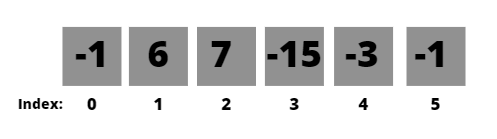For this given array, the output is 4.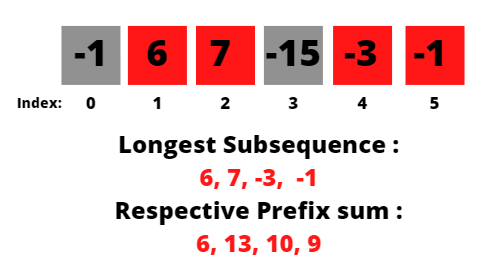Test Case 2: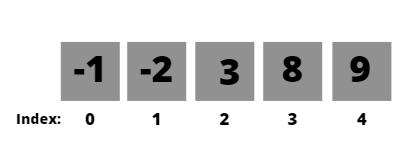For this given array, the output is 3.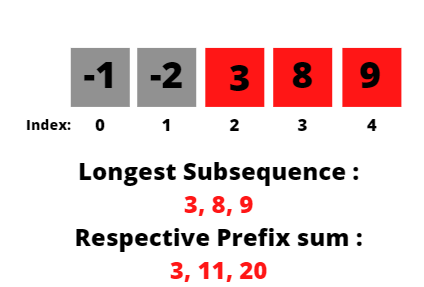NOTE: In this problem, we have to find the length of the longest subsequence, not the longest subarray. Subsequence refers to a non-contiguous sequence of a given sequence, obtained by deleting one or more elements from the original sequence.

## Approach

To solve this problem, we will use the Min Heap data structure. We will go through each element of the array and push it in the Min Heap. We will also add the element to the sum variable, which will keep track of the prefix sum of the current element.

If the prefix sum becomes negative, we subtract the most negative element from the heap and pop that element from the heap.

As it is a Min Heap, the most negative element will be present at the top of the heap. We are using Min Heap in this solution as it is very easy and quick to access the minimum element from any current step.

### Steps of Algorithm

1. Take input of vectors from the user.
2. Create an empty Min Heap using Priority Queue.
3. Initialize the sum variable as 0.
4. Run a for loop from 0 to n(size of vector)
5. Add the value of the current element of the vector to the sum
6. Add the current element of the vector to the Min Heap
7. Check if the sum has become negative
• If the sum is negative
• Subtract the most negative/top element from the sum
• Pop the top element from the Min Heap
8. Else
• Continue

## Implementation in C++

``````#include <bits/stdc++.h>
using namespace std;
int solve(vector<int> vec, int n)
{
priority_queue<int, vector<int>, greater<int>> min_heap; //Creating the Min Heap
int sum = 0;                                             //Initializing sum as 0
for (int i = 0; i < n; i++)
{
sum += vec[i];         //Adding current element's value to sum
min_heap.push(vec[i]); //Pushing current element to heap
if (sum < 0)           //If sum becomes negative
{
int a = min_heap.top();
sum -= a;       //Subtract top element's value from sum
min_heap.pop(); //Pop the top element from heap
}
}
return min_heap.size(); //return the ans
}
int main()
{
vector<int> vec;
int n, x;
cout << "Enter the size of the array:" << endl;
cin >> n;
cout << "Enter the elements:" << endl;
for (int i = 0; i < n; i++)
{
cin >> x;
vec.push_back(x);
}
cout << "Length of the longest subsequence is: " << solve(vec, n);
return 0;
}``````

Input:

``````Enter the size of the array:
6
Enter the elements:
-1 6 7 -15 -3 -1``````

Output:

``Length of the longest subsequence is: 4``

### Complexity Analysis

Time Complexity

O(n*logn) as we are traversing through a for loop ‘n’ number of times. The push and pop operations of priority queue take O(logn) time, making the overall time complexity O(n*logn).  Here, n refers to the number of elements in the input vector.

Space Complexity

O(n), as we are using a priority queue of size n. Here, n refers to the number of elements in the input vector.

### Dry Run of test cases

Let’s look at the dry run of Test Case 1 to understand the problem better.

The input array is,

-1, 6, 7, -15, -3, -1

Initially, sum = 0, and the Min Heap is also empty.

-1 is inserted to the Min Heap, and sum = -1.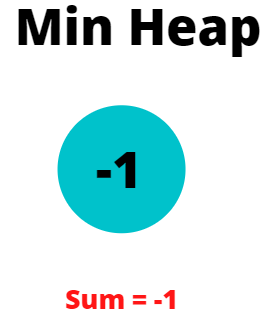But, as the sum becomes negative, we pop the top element from the Heap.

The next element, i.e., 6 is inserted in a heap, and the new sum becomes 6.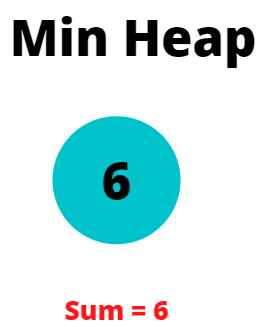We then go to the next element of the vector, i.e., 7. The current sum becomes 13 (6+7). Hence, 7 is also inserted into the heap.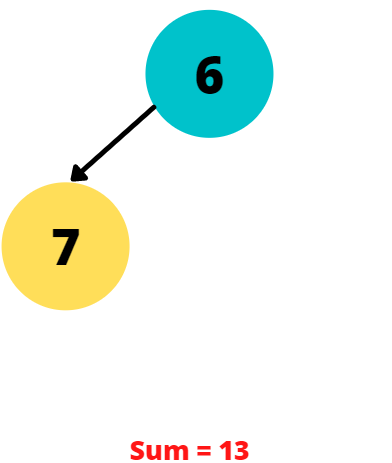The next element is -15, and adding it makes the sum negative.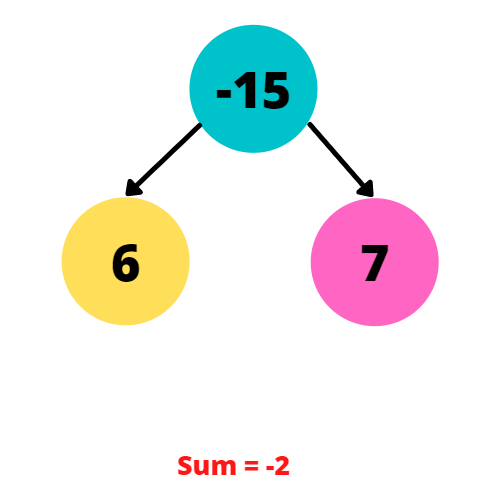The sum becomes -2, so we again pop the top element of the heap, i.e., -15.

We also deduct -15 from our sum, making sum = 13.

The next element in the vector is -3; adding it to our sum makes it 10, which is a positive number. So, it is also added to the Heap.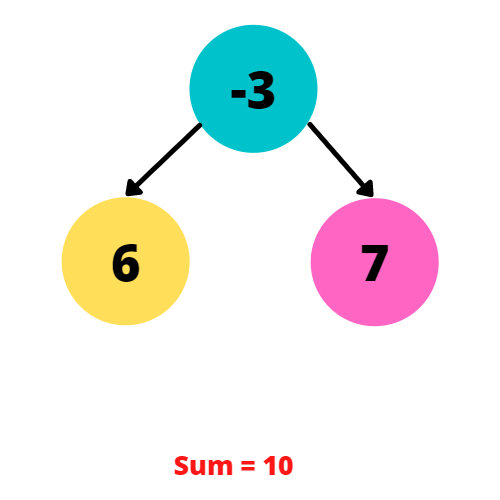We finally reach the last element of the vector, i.e., -1, and we add it to the current sum. It is also pushed into the Heap.

So our current sum is 9, and our Min Heap will look like this,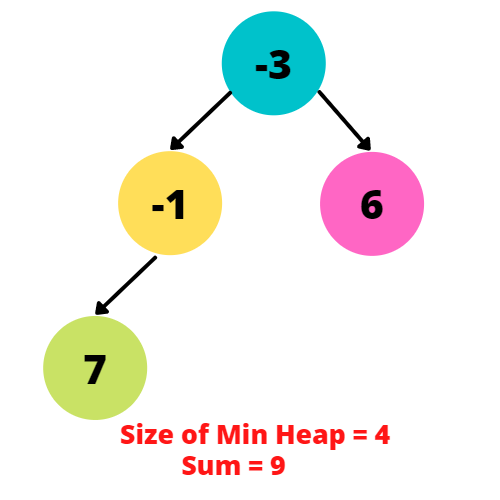In the end, the size of our Min Heap is 4, indicating the length of the longest subsequence.

Q1. What is Min Heap data structure?

Ans: Min Heap is a binary tree, where the root node of the tree has a value less than its children. Like the root node, the other internal nodes also need to have a smaller value than their respective children.

Q2. What is meant by the subsequence of an array?

Ans: Subsequence refers to a non-contiguous sequence of a given array, obtained by deleting one or more elements from the original array.

Q3. Is the longest subsequence the same as the longest subarray for a given array?

Ans: No, they are not the same. Longest subsequent refers to the longest possible non-contiguous sequence for a given array, but subarray refers to the longest possible contiguous section.

Q4. In which type of problems should we use Heap?

Ans: In problems where you have to find the minimum element (Min Heap) and maximum(Max Heap) element from a list of elements very quickly, Heap should be used.

## Key Takeaways

In this blog, we saw how to solve the given problem using Min Heap data structure.

We hope you completely understand the solution, but if you are new to Min Heap, we suggest you go through this blog to understand the critical concepts related to it.

Happy learning, Ninja!